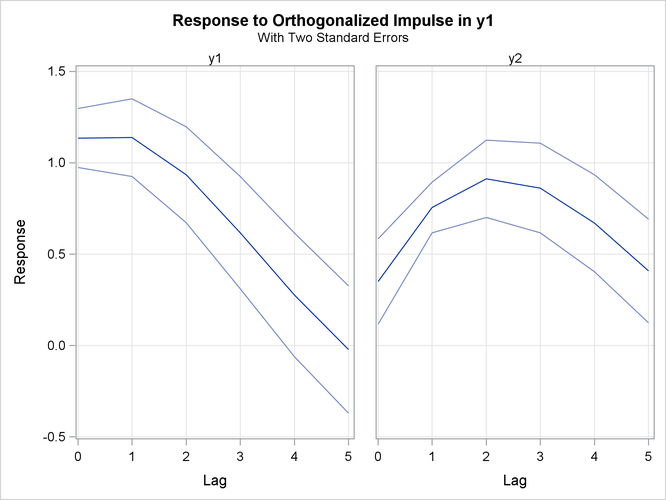The VARMAX Procedure

Impulse Response Function

Subsections:

Simple Impulse Response Function (IMPULSE=SIMPLE Option)

The VARMAX(p,q,s) model has a convergent representation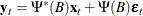where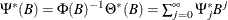and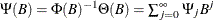.

The elements of the matrices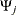from the operator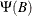, called the impulse response, can be interpreted as the impact that a shock in one variable has on another variable. Let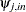be the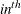element of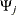at lag j, where i is the index for the impulse variable, and n is the index for the response variable (impulse
response). For instance,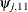is an impulse response to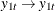, and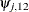is an impulse response to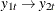.

Accumulated Impulse Response Function (IMPULSE=ACCUM Option)

The accumulated impulse response function is the cumulative sum of the impulse response function,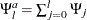.

Orthogonalized Impulse Response Function (IMPULSE=ORTH Option)

The MA representation of a VARMA(p,q) model with a standardized white noise innovation process offers another way to interpret a VARMA(p,q) model. Since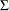is positive-definite, there is a lower triangular matrix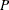such that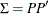. The alternate MA representation of a VARMA(p,q) model is written as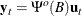where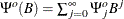,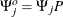, and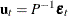.

The elements of the matrices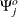, called the orthogonal impulse response, can be interpreted as the effects of the components of the standardized shock process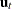on the process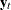at lag j.

Impulse Response of Transfer Function (IMPULSX=SIMPLE Option)

The coefficient matrix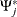from the transfer function operator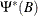can be interpreted as the effects that changes in the exogenous variables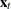have on the output variable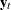at lag j; it is called an impulse response matrix in the transfer function.

Impulse Response of Transfer Function (IMPULSX=ACCUM Option)

The accumulated impulse response in the transfer function is the cumulative sum of the impulse response in the transfer function,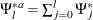.

The asymptotic distributions of the impulse functions can be seen in the section VAR and VARX Modeling.

The following statements provide the impulse response and the accumulated impulse response in the transfer function for a VARX(1,0) model.

proc varmax data=grunfeld plot=impulse;
model y1-y3 = x1 x2 / p=1 lagmax=5
printform=univariate
print=(impulsx=(all) estimates);
run;


In Figure 35.26, the variables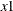and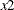are impulses and the variables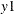,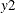, and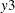are responses. You can read the table matching the pairs of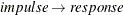such as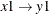,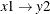,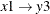,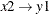,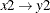, and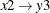. In the pair of, you can see the long-run responses ofto an impulse in(the values are 1.69281, 0.35399, 0.09090, and so on for lag 0, lag 1, lag 2, and so on, respectively).

Figure 35.26: Impulse Response in Transfer Function (IMPULSX= Option)

The VARMAX Procedure

Simple Impulse Response of Transfer Function
by Variable
Variable
Response\Impulse
Lag x1 x2
y1 0 1.69281 -0.00859
1 0.35399 0.01727
2 0.09090 0.00714
3 0.05136 0.00214
4 0.04717 0.00072
5 0.04620 0.00040
y2 0 -6.09850 2.57980
1 -5.15484 0.45445
2 -3.04168 0.04391
3 -2.23797 -0.01376
4 -1.98183 -0.01647
5 -1.87415 -0.01453
y3 0 -0.02317 -0.01274
1 1.57476 -0.01435
2 1.80231 0.00398
3 1.77024 0.01062
4 1.70435 0.01197
5 1.63913 0.01187

Figure 35.27 shows the responses of,, andto a forecast error impulse in.

Figure 35.27: Plot of Impulse Response in Transfer Function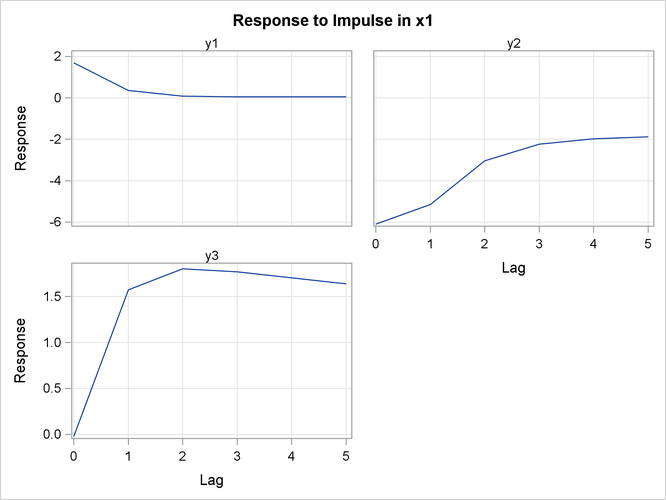Figure 35.28 shows the accumulated impulse response in transfer function.

Figure 35.28: Accumulated Impulse Response in Transfer Function (IMPULSX= Option)

Accumulated Impulse Response of Transfer
Function by Variable
Variable
Response\Impulse
Lag x1 x2
y1 0 1.69281 -0.00859
1 2.04680 0.00868
2 2.13770 0.01582
3 2.18906 0.01796
4 2.23623 0.01867
5 2.28243 0.01907
y2 0 -6.09850 2.57980
1 -11.25334 3.03425
2 -14.29502 3.07816
3 -16.53299 3.06440
4 -18.51482 3.04793
5 -20.38897 3.03340
y3 0 -0.02317 -0.01274
1 1.55159 -0.02709
2 3.35390 -0.02311
3 5.12414 -0.01249
4 6.82848 -0.00052
5 8.46762 0.01135

Figure 35.29 shows the accumulated responses of,, andto a forecast error impulse in.

Figure 35.29: Plot of Accumulated Impulse Response in Transfer Function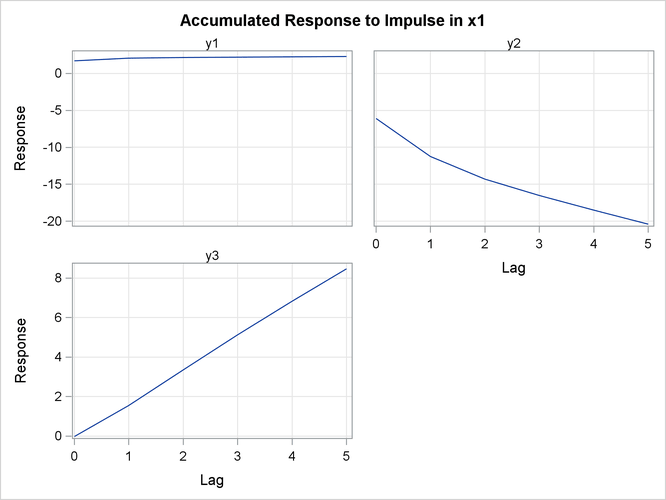The following statements provide the impulse response function, the accumulated impulse response function, and the orthogonalized impulse response function with their standard errors for a VAR(1) model. Parts of the VARMAX procedure output are shown in Figure 35.30, Figure 35.32, and Figure 35.34.

proc varmax data=simul1 plot=impulse;
model y1 y2 / p=1 noint lagmax=5
print=(impulse=(all))
printform=univariate;
run;


Figure 35.30 is the output in a univariate format associated with the PRINT=(IMPULSE=) option for the impulse response function. The keyword STD stands for the standard errors of the elements. The matrix in terms of the lag 0 does not print since it is the identity. In Figure 35.30, the variablesandof the first row are impulses, and the variablesandof the first column are responses. You can read the table matching thepairs, such as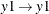,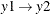,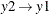, and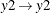. For example, in the pair ofat lag 3, the response is 0.8055. This represents the impact on y1 of one-unit change inafter 3 periods. As the lag gets higher, you can see the long-run responses ofto an impulse in itself.

Figure 35.30: Impulse Response Function (IMPULSE= Option)

The VARMAX Procedure

Simple Impulse Response by Variable
Variable
Response\Impulse
Lag y1 y2
y1 1 1.15977 -0.51058
STD 0.05508 0.05898
2 1.06612 -0.78872
STD 0.10450 0.10702
3 0.80555 -0.84798
STD 0.14522 0.14121
4 0.47097 -0.73776
STD 0.17191 0.15864
5 0.14315 -0.52450
STD 0.18214 0.16115
y2 1 0.54634 0.38499
STD 0.05779 0.06188
2 0.84396 -0.13073
STD 0.08481 0.08556
3 0.90738 -0.48124
STD 0.10307 0.09865
4 0.78943 -0.64856
STD 0.12318 0.11661
5 0.56123 -0.65275
STD 0.14236 0.13482

Figure 35.31 shows the responses ofandto a forecast error impulse inwith two standard errors.

Figure 35.31: Plot of Impulse Response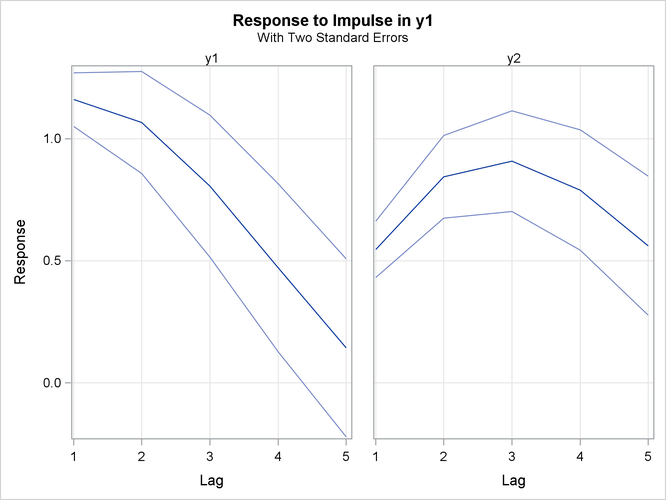Figure 35.32 is the output in a univariate format associated with the PRINT=(IMPULSE=) option for the accumulated impulse response function. The matrix in terms of the lag 0 does not print since it is the identity.

Figure 35.32: Accumulated Impulse Response Function (IMPULSE= Option)

Accumulated Impulse Response by Variable
Variable
Response\Impulse
Lag y1 y2
y1 1 2.15977 -0.51058
STD 0.05508 0.05898
2 3.22589 -1.29929
STD 0.21684 0.22776
3 4.03144 -2.14728
STD 0.52217 0.53649
4 4.50241 -2.88504
STD 0.96922 0.97088
5 4.64556 -3.40953
STD 1.51137 1.47122
y2 1 0.54634 1.38499
STD 0.05779 0.06188
2 1.39030 1.25426
STD 0.17614 0.18392
3 2.29768 0.77302
STD 0.36166 0.36874
4 3.08711 0.12447
STD 0.65129 0.65333
5 3.64834 -0.52829
STD 1.07510 1.06309

Figure 35.33 shows the accumulated responses ofandto a forecast error impulse inwith two standard errors.

Figure 35.33: Plot of Accumulated Impulse Response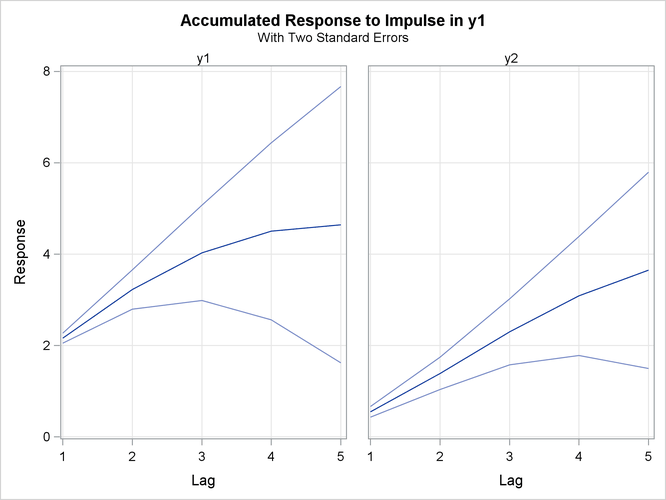Figure 35.34 is the output in a univariate format associated with the PRINT=(IMPULSE=) option for the orthogonalized impulse response function. The two right-hand side columns,and, represent the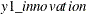and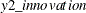variables. These are the impulses variables. The left-hand side column contains responses variables,and. You can read the table by matching thepairs such as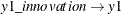,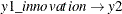,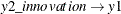, and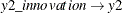.

Figure 35.34: Orthogonalized Impulse Response Function (IMPULSE= Option)

Orthogonalized Impulse Response by Variable
Variable
Response\Impulse
Lag y1 y2
y1 0 1.13523 0.00000
STD 0.08068 0.00000
1 1.13783 -0.58120
STD 0.10666 0.14110
2 0.93412 -0.89782
STD 0.13113 0.16776
3 0.61756 -0.96528
STD 0.15348 0.18595
4 0.27633 -0.83981
STD 0.16940 0.19230
5 -0.02115 -0.59705
STD 0.17432 0.18830
y2 0 0.35016 1.13832
STD 0.11676 0.08855
1 0.75503 0.43824
STD 0.06949 0.10937
2 0.91231 -0.14881
STD 0.10553 0.13565
3 0.86158 -0.54780
STD 0.12266 0.14825
4 0.66909 -0.73827
STD 0.13305 0.15846
5 0.40856 -0.74304
STD 0.14189 0.16765

In Figure 35.4, there is a positive correlation between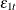and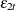. Therefore, shock incan be accompanied by a shock inin the same period. For example, in the pair of, you can see the long-run responses ofto an impulse in.

Figure 35.35 shows the orthogonalized responses ofandto a forecast error impulse inwith two standard errors.

Figure 35.35: Plot of Orthogonalized Impulse Response